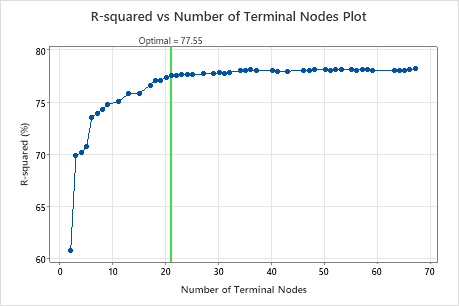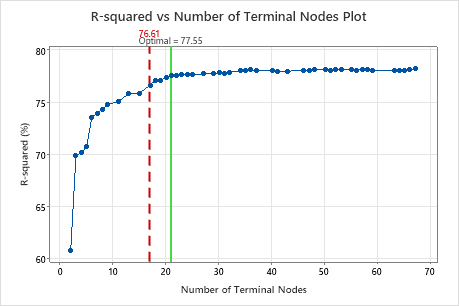# R-squared vs number of terminal nodes plot for CART® Regression

Minitab displays a plot of a R2 value against the number of terminal nodes in the tree so that you can select a tree to evaluate further. If you use a test data set or k-fold cross-validation to validate the performance of the tree, then the R2 value is for the validation data.

The R-squared vs Number of Terminal Nodes Plot displays the R2 value for each tree. By default, the initial regression tree is the smallest tree with an R2 value within 1 standard error of the value for the maximum R2 value. When the analysis uses cross-validation or a test data set, the R2 value is from the validation sample. The values for the validation sample typically level off and eventually start to decline as the tree grows larger.

Click Select an Alternative Tree to open an interactive plot that includes a table of model summary statistics. Use the plot to investigate alternative trees with similar performance.

Typically, you select an alternative tree for one of the following two reasons:
• The tree that Minitab selects is part of a pattern where the criterion improves. One or more trees that have a few more nodes are part of the same pattern. Typically, you want to make predictions from a tree with as much prediction accuracy as possible.
• The tree that Minitab selects is part of a pattern where the criterion is relatively flat. One or more trees with similar model summary statistics have much fewer nodes than the optimal tree. Typically, a tree with fewer terminal nodes gives a clearer picture of how each predictor variable affects the response values. A smaller tree also makes it easier to identify a few target groups for further studies. If the difference in prediction accuracy for a smaller tree is negligible, you can also use the smaller tree to evaluate the relationships between the response and the predictor variables

## InterpretationBy using this site you agree to the use of cookies for analytics and personalized content.  Read our policy